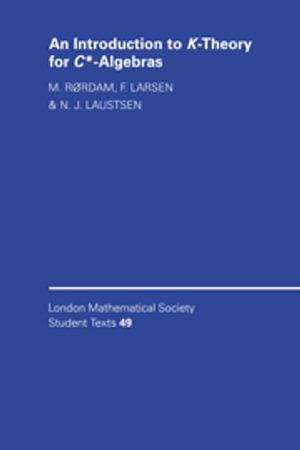+612 9045 4394CHECKOUT# An Introduction to K-Theory for C*-Algebras

### London Mathematical Society Student Texts, 49

Paperback Published: 15th September 2000
ISBN: 9780521789448
Number Of Pages: 256

### Paperback

RRP \$69.95
\$62.75
10%
OFF
Ships in 10 to 15 business days

Earn 126 Qantas Points
on this Book

## Other Available Editions(Hide)

Over the last 25 years K-theory has become an integrated part of the study of C*-algebras. This book gives an elementary introduction to this interesting and rapidly growing area of mathematics. Fundamental to K-theory is the association of a pair of Abelian groups, K0(A) and K1(A), to each C*-algebra A. These groups reflect the properties of A in many ways. This book covers the basic properties of the functors K0 and K1 and their interrelationship. Applications of the theory include Elliott's classification theorem for AF-algebras, and it is shown that each pair of countable Abelian groups arises as the K-groups of some C*-algebra. The theory is well illustrated with 120 exercises and examples, making the book ideal for beginning graduate students working in functional analysis, especially operator algebras, and for researchers from other areas of mathematics who want to learn about this subject.

#### Industry Reviews

'The textbook is a nice introduction to the subject preparing the ground for the study of more advanced texts.' H. Schroeder, Zentralblatt fur Mathematik

 Preface p. ix C*-Algebra Theory p. 1 C*-algebras and *-homomorphisms p. 1 Spectral theory p. 5 Matrix algebras p. 9 Exercises p. 10 Projections and Unitary Elements p. 15 Homotopy classes of unitary elements p. 15 Equivalence of projections p. 21 Semigroups of projections p. 28 Exercises p. 30 The K[subscript 0]-Group of a Unital C*-Algebra p. 35 Definition of the K[subscript 0]-group of a unital C*-algebra p. 35 Functoriality of K[subscript 0] p. 41 Examples p. 46 Exercises p. 54 The Functor K[subscript 0] p. 59 Definition and functoriality of K[subscript 0] p. 59 The standard picture of the group K[subscript 0](A) p. 62 Half and split exactness and stability of K[subscript 0] p. 66 Exercises p. 70 The Ordered Abelian Group K[subscript 0](A) p. 77 The ordered K[subscript 0]-group of stably finite C*-algebras p. 77 States on K[subscript 0](A) and traces on A p. 82 Exercises p. 83 Inductive Limit C*-Algebras p. 89 Products and sums of C*-algebras p. 89 Inductive limits p. 91 Continuity of K[subscript 0] p. 97 Stabilized C*-algebras p. 101 Exercises p. 104 Classification of AF-Algebras p. 109 Finite dimensional C*-algebras p. 109 AF-algebras p. 113 Elliott's classification theorem p. 118 UHF-algebras p. 125 Exercises p. 130 The Functor K[subscript 1] p. 133 Definition of the K[subscript 1]-group p. 133 Functoriality of K[subscript 1] p. 138 K[subscript 1]-groups and determinants p. 144 Exercises p. 147 The Index Map p. 153 Definition of the index map p. 154 The index map and partial isometries p. 158 An exact sequence of K-groups p. 163 Fredholm operators and Fredholm index p. 165 Exercises p. 169 The Higher K-Functors p. 175 The isomorphism between K[subscript 1](A) and K[subscript 0](SA) p. 175 The long exact sequence in K-theory p. 178 Exercises p. 182 Bott Periodicity p. 185 The Bott map p. 185 The proof of Bott periodicity p. 187 Applications of Bott periodicity p. 199 Homotopy groups and K-theory p. 201 The holomorphic function calculus p. 204 Exercises p. 206 The Six-Term Exact Sequence p. 209 The exponential map and the six-term exact sequence p. 209 An explicit description of the exponential map p. 211 Exercises p. 215 Inductive Limits of Dimension Drop Algebras p. 219 Dimension drop algebras p. 219 Countable Abelian groups as K-groups p. 222 Exercises p. 228 References p. 231 Table of K-groups p. 234 Index of symbols p. 236 General index p. 239 Table of Contents provided by Syndetics. All Rights Reserved.

ISBN: 9780521789448
ISBN-10: 0521789443
Series: London Mathematical Society Student Texts, 49
Audience: Professional
Format: Paperback
Language: English
Number Of Pages: 256
Published: 15th September 2000
Publisher: CAMBRIDGE UNIV PR
Country of Publication: GB
Dimensions (cm): 21.59 x 14.61  x 1.27
Weight (kg): 0.39

Earn 126 Qantas Points
on this Book